# Pipe network analysis with examples

Mar. 16, 2015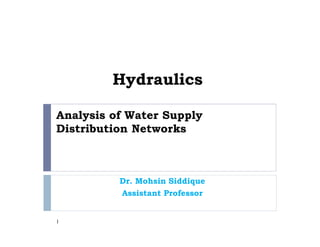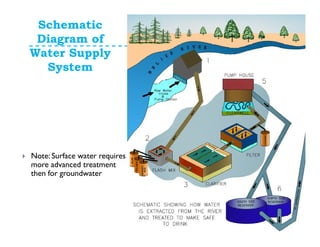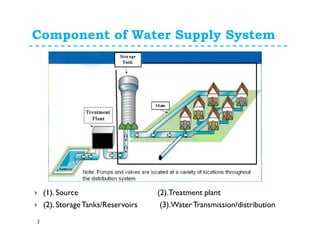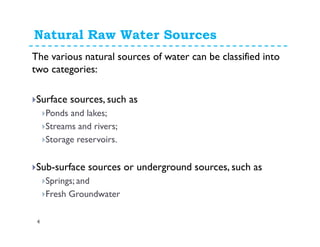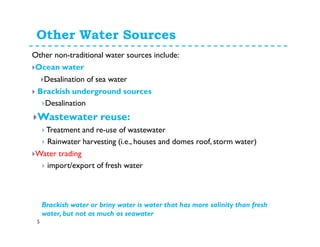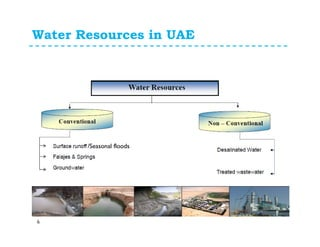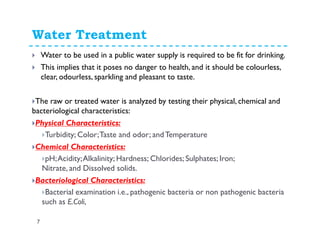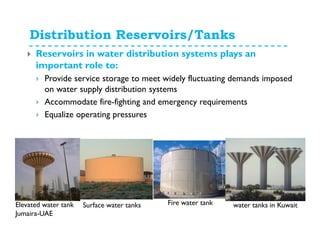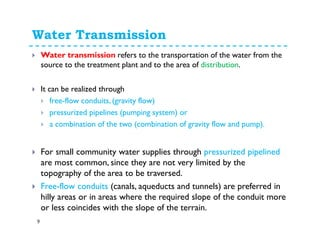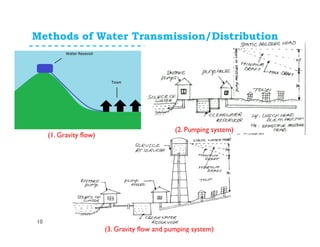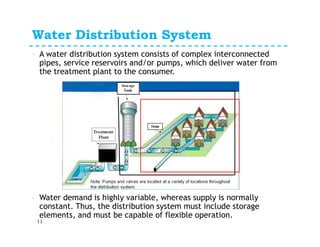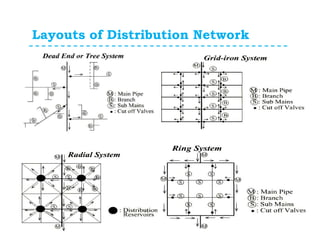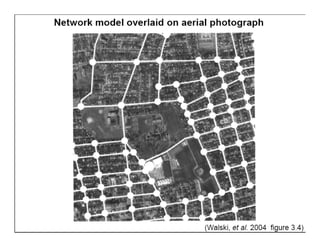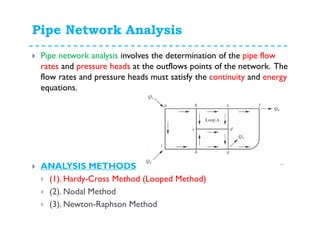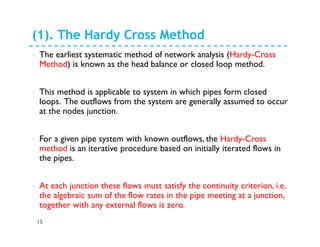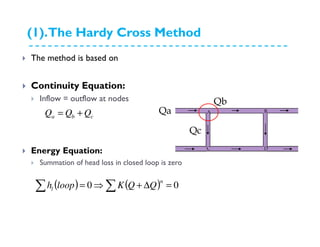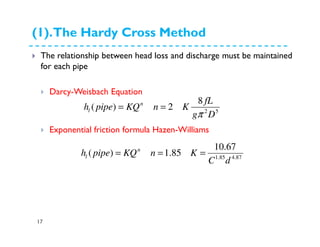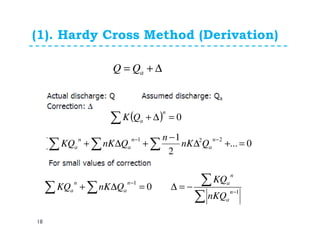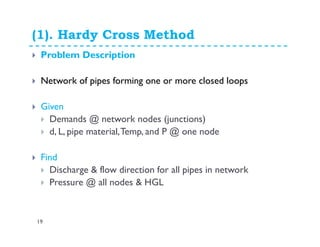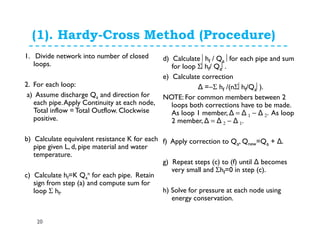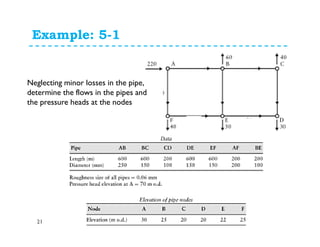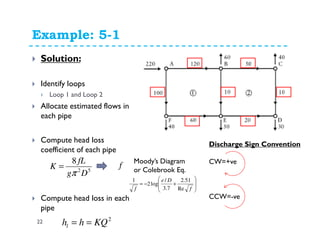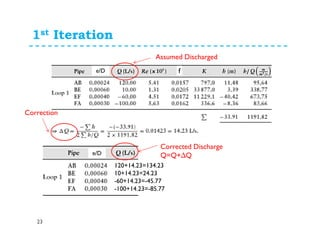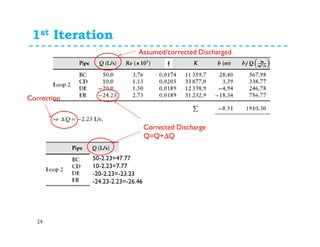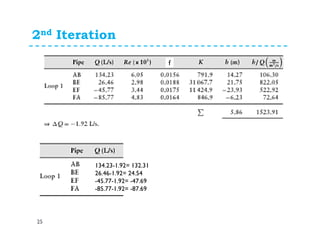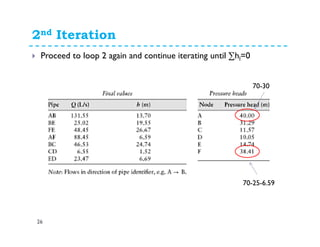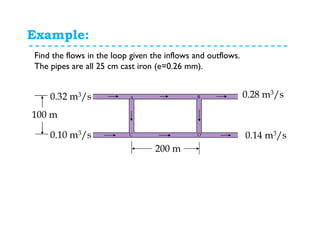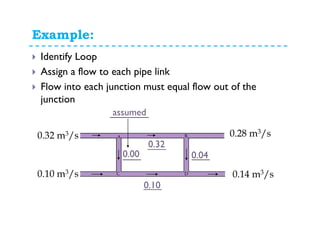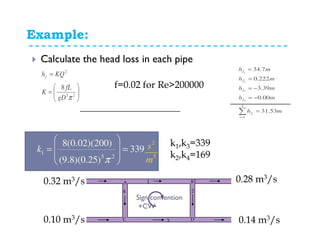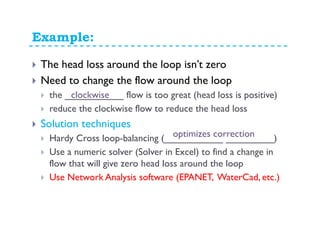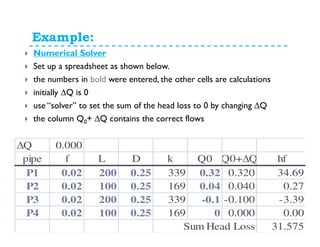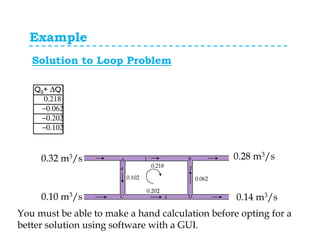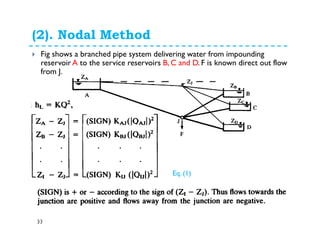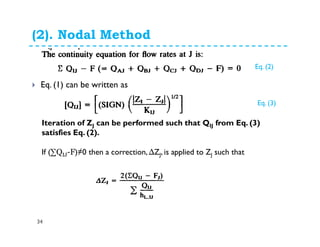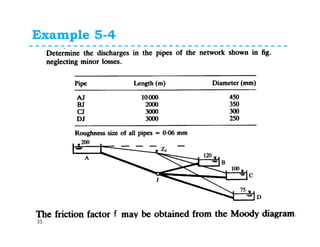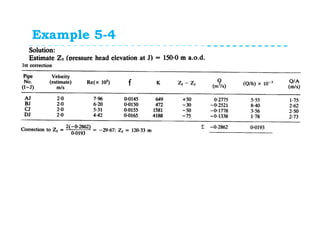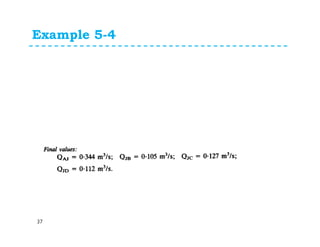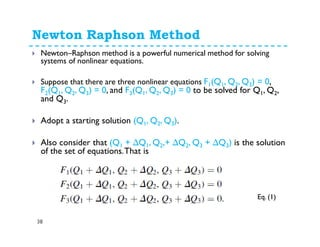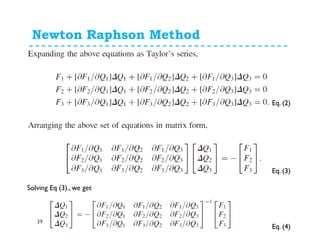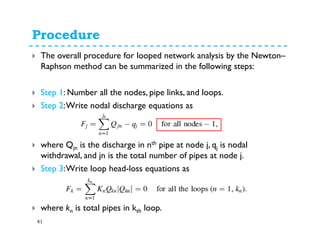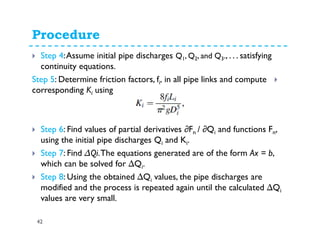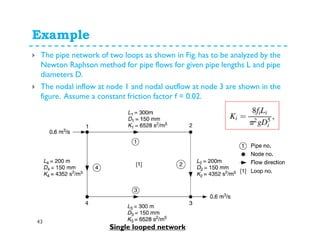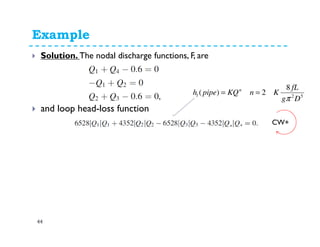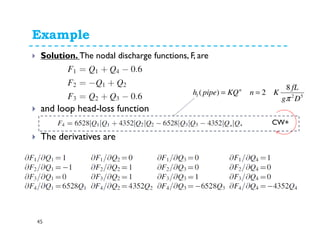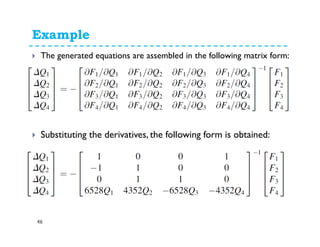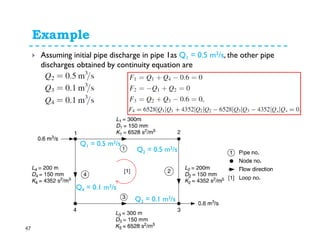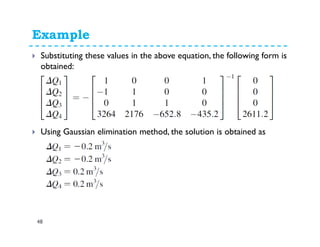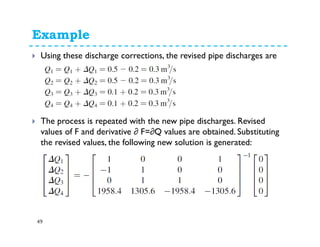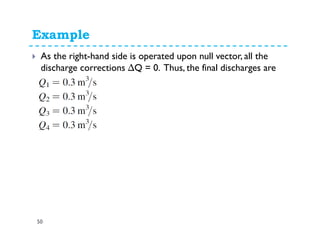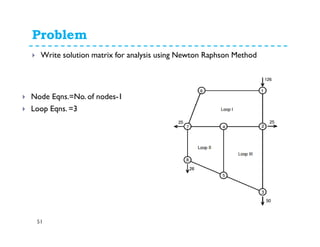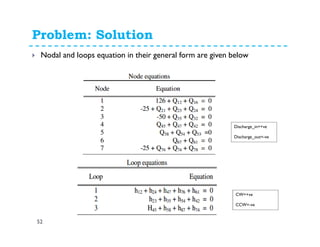1 of 54

### Pipe network analysis with examples

• 1. Analysis of Water Supply Distribution Networks Dr. Mohsin Siddique Assistant Professor 1
• 2. Schematic Diagram of Water Supply System Note: Surface water requires more advanced treatment then for groundwater
• 3. Component of Water Supply System 3 (1). Source (2).Treatment plant (2). StorageTanks/Reservoirs (3).WaterTransmission/distribution
• 4. The various natural sources of water can be classified into two categories: Surface sources, such as Ponds and lakes; Streams and rivers; Storage reservoirs. Sub-surface sources or underground sources, such as Springs; and Fresh groundwater Natural Raw Water Sources 4
• 5. Other non-traditional water sources include: Ocean water Desalination of sea water Brackish underground sources Desalination Wastewater reuse Treatment and re-use of wastewater Rainwater harvesting (i.e., houses and domes roof, storm water) Water trading !! import/export of fresh water Virtual water trade Other Water Sources Brackish water or briny water is water that has more salinity than fresh water, but not as much as seawater 5
• 6. Water Resources in UAE 6 /Seasonal floods
• 7. Water Quality and Treatment 7 Water to be used in a public water supply is required to be fit for drinking. This implies that it poses no danger to health, and it should be colourless, clear, odourless, sparkling and pleasant to taste. The raw or treated water is analyzed by testing their physical, chemical and bacteriological characteristics: Physical Characteristics: Turbidity; Color;Taste and odor; andTemperature Chemical Characteristics: pH;Acidity;Alkalinity; Hardness; Chlorides; Sulphates; Iron; Nitrate, and Dissolved solids. Bacteriological Characteristics: Bacterial examination i.e., pathogenic bacteria or non pathogenic bacteria such as E.Coli,
• 8. Distribution Reservoirs/Tanks Reservoirs in water distribution systems plays an important role to: Provide service storage to meet widely fluctuating demands imposed on water supply distribution systems Accommodate fire-fighting and emergency requirements Equalize operating pressures Fire water tankElevated water tank Jumaira-UAE Surface water tanks water tanks in Kuwait
• 9. Water Transmission 9 Water transmission refers to the transportation of the water from the source to the treatment plant and to the area of distribution. It can be realized through free-flow conduits, (gravity flow) pressurized pipelines (pumping system) or a combination of the two (combination of gravity flow and pump). For small community water supplies through pressurized pipelined are most common, since they are not very limited by the topography of the area to be traversed. Free-flow conduits (canals, aqueducts and tunnels) are preferred in hilly areas or in areas where the required slope of the conduit more or less coincides with the slope of the terrain.
• 10. Methods of Water Transmission/Distribution 10 (pumping system) (1. Gravity flow) (2. Pumping system) (3. Gravity flow and pumping system)
• 11. Water Distribution System 11 A water distribution system consists of complex interconnected pipes, service reservoirs and/or pumps, which deliver water from the treatment plant to the consumer. Water demand is highly variable, whereas supply is normally constant. Thus, the distribution system must include storage elements, and must be capable of flexible operation.
• 13. Design of Water Supply Networks General Consideration: A municipal water distribution system includes a network of mains with storage reservoirs, booster pumping stations (if needed),fire hydrants, and service lines.Arterial mains, or feeders, are pipelines of larger size that are connected to the transmission lines that supply the water for distribution.A major water demand areas in a city should be served by a feeder loop; where possible the arterial mains should be laid in duplicate. Parallel feeder mains are cross connected at intervals of one to two kilometers, with valves to permit isolation of sections in case of a main break. Distribution lines tie to each arterial loop, forming a complete gridiron “close loops” system that domestic, commercial consumers and services fire hydrants.The gridiron system illustrated in the Figure 1 is the best arrangement for distributing water.All of the arterials and secondary mains are looped and interconnected, eliminating dead ends and permitting water circulation such that a heavy discharge from one main allows drawing water from other pipes. The dead-end system shown in Figure 2 is avoided in new construction and can often be corrected in existing systems by proper looping.Trunk lines placed in the main streets supply sub-mains, which are extended at right angles to serve individual streets without interconnections. Consequently, if a pipe break occurs substantial portion of the community may be without water. Under a some conditions, the water in dead-end lines develops tastes and odors from stagnation.To prevent this, dead ends may require frequent flushing where houses are widely separated.
• 14. Pipe Network Analysis Pipe network analysis involves the determination of the pipe flow rates and pressure heads at the outflows points of the network. The flow rates and pressure heads must satisfy the continuity and energy equations. ANALYSIS METHODS (1). Hardy-Cross Method (Looped Method) (2). Nodal Method (3). Newton-Raphson Method
• 15. (1). The Hardy Cross Method 15 The earliest systematic method of network analysis (Hardy-Cross Method) is known as the head balance or closed loop method. This method is applicable to system in which pipes form closed loops. The outflows from the system are generally assumed to occur at the nodes junction. For a given pipe system with known outflows, the Hardy-Cross method is an iterative procedure based on initially iterated flows in the pipes. At each junction these flows must satisfy the continuity criterion, i.e. the algebraic sum of the flow rates in the pipe meeting at a junction, together with any external flows is zero.
• 16. (1).The Hardy Cross Method The method is based on Continuity Equation: Inflow = outflow at nodes Energy Equation: Summation of head loss in closed loop is zero cba QQQ += ( ) ( )∑ ∑ =∆+⇒= 00 n l QQKlooph A B C D Qa Qb Qc
• 17. (1).The Hardy Cross Method 17 The relationship between head loss and discharge must be maintained for each pipe Darcy-Weisbach Equation Exponential friction formula Hazen-Williams 52 8 2)( Dg fL KnKQpipeh n l π === 87.485.1 67.10 85.1)( dC KnKQpipeh n l ===
• 18. (1). Hardy Cross Method (Derivation) 18 QQQ a ∆+= ( ) 0=∆+∑ n a QQK ( ) ( ) 0... 2 1 221 =+∆ − +∆+∑ ∑∑ −− n a n a n a QQnK n QQnKKQ ( ) ∑ ∑∑ ∑ − − −=∆=∆+ 1 1 0 n a n an a n a nKQ KQ QQQnKKQ
• 19. (1). Hardy Cross Method 19 Problem Description Network of pipes forming one or more closed loops Given Demands @ network nodes (junctions) d, L, pipe material,Temp, and P @ one node Find Discharge & flow direction for all pipes in network Pressure @ all nodes & HGL
• 20. (1). Hardy-Cross Method (Procedure) 20 1. Divide network into number of closed loops. 2. For each loop: a) Assume discharge Qa and direction for each pipe.Apply Continuity at each node, Total inflow =Total Outflow. Clockwise positive. b) Calculate equivalent resistance K for each pipe given L, d, pipe material and water temperature. c) Calculate hf=K Qa n for each pipe. Retain sign from step (a) and compute sum for loop Σ hf. d) Calculate hf / Qa for each pipe and sum for loop Σhf/ Qa. e) Calculate correction ∆Q = −Σ hf /(nΣhf/Qa). NOTE: For common members between 2 loops both corrections have to be made. As loop 1 member, ∆Q = ∆Q 1 − ∆Q 2. As loop 2 member, ∆ Q = ∆ Q 2 − ∆ Q 1. f) Apply correction to Qa, Qnew=Qa + ∆ Q. g) Repeat steps (c) to (f) until ∆Q becomes very small and Σhf=0 in step (c). h) Solve for pressure at each node using energy conservation.
• 21. Example: 5-1 21 Neglecting minor losses in the pipe, determine the flows in the pipes and the pressure heads at the nodes (kinematic viscosity= 1.13x10-6m2/s)
• 22. Example: 5-1 22 Solution: Identify loops Loop 1 and Loop 2 Allocate estimated flows in each pipe Compute head loss coefficient of each pipe Compute head loss in each pipe Discharge Sign Convention CW=+ve CCW=-ve 52 8 Dg fL K π = 2 KQhhl == f Moody’s Diagram or Colebrook Eq.         +−= f De f Re 51.2 7.3 / log2 1
• 25. 2nd Iteration 25 134.23-1.92= 132.31 26.46-1.92= 24.54 -45.77-1.92= -47.69 -85.77-1.92= -87.69 f hL hL/Q
• 26. 2nd Iteration 26 Proceed to loop 2 again and continue iterating until ∑hl=0 70-25-6.59 70-30
• 27. Example: Find the flows in the loop given the inflows and outflows. The pipes are all 25 cm cast iron (e=0.26 mm). A B C D0.10 m3/s 0.32 m3/s 0.28 m3/s 0.14 m3/s 200 m 100 m
• 28. Example: Identify Loop Assign a flow to each pipe link Flow into each junction must equal flow out of the junction A B C D0.10 m3/s 0.32 m3/s 0.28 m3/s 0.14 m3/s 0.32 0.00 0.10 0.04 assumed
• 29. Example: Calculate the head loss in each pipe f=0.02 for Re>200000       = = 25 2 8 πgD fL K KQhf 339 )25.0)(8.9( )200)(02.0(8 25 1 =         = π k k1,k3=339 k2,k4=169 A B C D0.10 m3/s 0.32 m3/s 0.28 m3/s 0.14 m3/s 1 4 2 3 mh mh mh mh mh i f f f f f i 53.31 00.0 39.3 222.0 7.34 4 1 4 3 2 1 = −= −= = = ∑= Sign convention +CW 2 5 s m
• 30. Example: The head loss around the loop isn’t zero Need to change the flow around the loop the ___________ flow is too great (head loss is positive) reduce the clockwise flow to reduce the head loss Solution techniques Hardy Cross loop-balancing (___________ _________) Use a numeric solver (Solver in Excel) to find a change in flow that will give zero head loss around the loop Use Network Analysis software (EPANET, WaterCad, etc.) clockwise optimizes correction
• 31. Example: Numerical Solver Set up a spreadsheet as shown below. the numbers in bold were entered, the other cells are calculations initially ∆Q is 0 use “solver” to set the sum of the head loss to 0 by changing ∆Q the column Q0+ ∆Q contains the correct flows ∆Q 0.000 pipe f L D k Q0 Q0+∆Q hf P1 0.02 200 0.25 339 0.32 0.320 34.69 P2 0.02 100 0.25 169 0.04 0.040 0.27 P3 0.02 200 0.25 339 -0.1 -0.100 -3.39 P4 0.02 100 0.25 169 0 0.000 0.00 31.575Sum Head Loss
• 32. Example A B C D0.10 m3/s 0.32 m3/s 0.28 m3/s 0.14 m3/s 0.218 0.102 0.202 0.062 1 4 2 3 Q0+ ∆Q 0.218 −0.062 −0.202 −0.102 You must be able to make a hand calculation before opting for a better solution using software with a GUI. Solution to Loop Problem
• 33. (2). Nodal Method 33 Fig shows a branched pipe system delivering water from impounding reservoir A to the service reservoirs B, C and D. F is known direct out flow from J. Eq. (1)
• 34. (2). Nodal Method 34 Eq. (1) can be written as Eq. (2) Eq. (3) Iteration of ZJ can be performed such that QIJ from Eq. (3) satisfies Eq. (2). If (∑QIJ-F)≠0 then a correction, ∆ZJ, is applied to ZJ such that
• 38. Newton Raphson Method 38 Newton–Raphson method is a powerful numerical method for solving systems of nonlinear equations. Suppose that there are three nonlinear equations F1(Q1, Q2, Q3) = 0, F2(Q1, Q2, Q3) = 0, and F3(Q1, Q2, Q3) = 0 to be solved for Q1, Q2, and Q3. Adopt a starting solution (Q1, Q2, Q3). Also consider that (Q1 + ∆Q1, Q2,+ ∆Q2, Q3 + ∆Q3) is the solution of the set of equations.That is Eq. (1)
• 39. Newton Raphson Method 39 Eq. (2) Eq. (3) Solving Eq (3)., we get Eq. (4)
• 41. Procedure 41 The overall procedure for looped network analysis by the Newton– Raphson method can be summarized in the following steps: Step 1: Number all the nodes, pipe links, and loops. Step 2:Write nodal discharge equations as where Qjn is the discharge in nth pipe at node j, qj is nodal withdrawal, and jn is the total number of pipes at node j. Step 3:Write loop head-loss equations as where kn is total pipes in kth loop.
• 42. Procedure 42 Step 4:Assume initial pipe discharges Q1, Q2, and Q3., . . . satisfying continuity equations. in all pipe links and compute,ifDetermine friction factors,:5Step usingiKcorresponding Step 6: Find values of partial derivatives ∂Fn / ∂Qi and functions Fn, using the initial pipe discharges Qi and Ki. Step 7: Find ∆Qi.The equations generated are of the form Ax = b, which can be solved for ∆Qi. Step 8: Using the obtained ∆Qi values, the pipe discharges are modified and the process is repeated again until the calculated ∆Qi values are very small.
• 43. Example 43 The pipe network of two loops as shown in Fig. has to be analyzed by the Newton Raphson method for pipe flows for given pipe lengths L and pipe diameters D. The nodal inflow at node 1 and nodal outflow at node 3 are shown in the figure. Assume a constant friction factor f = 0.02. Single looped network
• 44. Example 44 Solution: Write nodal and loop equations Node1: Q1+Q4-0.6=0 Node 2: Q1+Q2=0 Node 3: Q2+Q3-0.6=0 Node 4: !!! and loop Eq. K1Q1 2+ K2Q2 2+ K3Q3 2+ K4Q4 2=0 Q1 Q2 Q4 Q3 52 8 2 )( Dg fL Kn KQpipeh n L π = =
• 45. Example 45 Assume initial pipe discharges based on continuity euqtion: Q1 = 0.5 m3/s Apply signs to flowrate in eqns Nodal discharge functions, F, are Q1+Q4-0.6=F1 - Q1+Q2=F2 Q2+Q3-0.6=F3 Loop head-loss function K1Q1 2+ K2Q2 2 - K3Q3 2 - K4Q4 2=F4 In = + Out = -ve = 0.5 0.5 0.1 0.1 Apply signs (+ or -)
• 46. Example 46 The final nodal discharge functions, F, become and loop head-loss function is F4=6528Q1 2+ 4352Q2 2 - 6528Q3 2 - 4352Q4 2=0 Take derivatives of equations:
• 47. Example 47 Assembled equation in the following matrix form: Substitute the derivatives
• 48. Example 48 Now, calculate values of F1 to F4 using previously assumed flows i.e., Q1 = 0.5 m3/s F4=6528Q1 2+ 4352Q2 2 - 6528Q3 2 - 4352Q4 2=2611.2
• 49. Example 49 Substitute values of F1 to F4 and Q1 to Q4 in the matrix form : Solve the matrix, we get following flowrate corrections:
• 50. Example 50 Using discharge corrections, the revised pipe flowrates become: The process is repeated with the new pipe flowrates. Revised values of F and derivative ∂F/∂Q values are obtained. Substituting the revised values, the following new solution matrix is generated:
• 51. Example 51 As the right-hand side is operated upon null vector, all the discharge corrections ∆Q = 0. Thus, the final flowrates are
• 52. Problem 52 Write solution matrix for analysis using Newton Raphson Method Node Eqns.=No. of nodes-1 Loop Eqns. =3
• 53. Problem: Solution 53 Nodal and loops equation in their general form are given below Discharge_in=+ve Discharge_out=-ve CW=+ve CCW=-ve